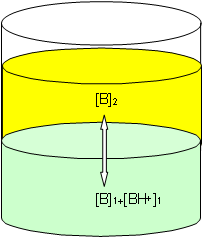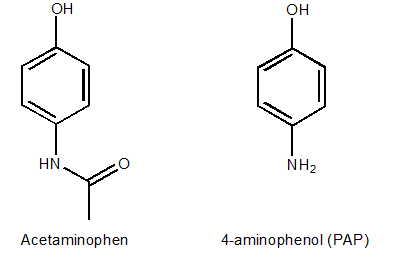# Liquid-Liquid Extraction

$$\newcommand{\vecs}{\overset { \rightharpoonup} {\mathbf{#1}} }$$ $$\newcommand{\vecd}{\overset{-\!-\!\rightharpoonup}{\vphantom{a}\smash {#1}}}$$$$\newcommand{\id}{\mathrm{id}}$$ $$\newcommand{\Span}{\mathrm{span}}$$ $$\newcommand{\kernel}{\mathrm{null}\,}$$ $$\newcommand{\range}{\mathrm{range}\,}$$ $$\newcommand{\RealPart}{\mathrm{Re}}$$ $$\newcommand{\ImaginaryPart}{\mathrm{Im}}$$ $$\newcommand{\Argument}{\mathrm{Arg}}$$ $$\newcommand{\norm}{\| #1 \|}$$ $$\newcommand{\inner}{\langle #1, #2 \rangle}$$ $$\newcommand{\Span}{\mathrm{span}}$$ $$\newcommand{\id}{\mathrm{id}}$$ $$\newcommand{\Span}{\mathrm{span}}$$ $$\newcommand{\kernel}{\mathrm{null}\,}$$ $$\newcommand{\range}{\mathrm{range}\,}$$ $$\newcommand{\RealPart}{\mathrm{Re}}$$ $$\newcommand{\ImaginaryPart}{\mathrm{Im}}$$ $$\newcommand{\Argument}{\mathrm{Arg}}$$ $$\newcommand{\norm}{\| #1 \|}$$ $$\newcommand{\inner}{\langle #1, #2 \rangle}$$ $$\newcommand{\Span}{\mathrm{span}}$$$$\newcommand{\AA}{\unicode[.8,0]{x212B}}$$

## Liquid-liquid extraction (LLE)

Liquid-liquid extraction (LLE) is based on the transfer of a solute from one liquid phase into another immiscible liquid phase according to differences in solubility. A common analytical challenge is measuring the level of a dilute analyte in a complex aqueous sample matrix, for example blood plasma or wastewater. Extraction of the analyte (A) from the aqueous sample into an immiscible organic solvent can both reduce matrix interferences and facilitate concentration of the analyte. When two immiscible phases are in contact, an analyte (A) that is soluble in the two phases will exchange between them. In this system, phase 1 could be blood plasma and phase 2 an immiscible solvent like dichloromethane. The equilibrium concentrations of A in the two phases will depend upon its relative solubility in each phase defined by the chemical equilibrium where [A]1 represents the concentration of species A in phase 1 and [A]2 represents the concentration of A in phase 2:

$\mathrm{\color{firebrick}{[A]_1} \color{black}\leftarrow \rightarrow \color{navy}{[A]_2}}\nonumber$

The analyte is said to partition between the two phases and the equilibrium constant for this reaction is called the partition coefficient, KP or P.

$\mathrm{K_P = P= \dfrac{\color{navy}[A]_2}{\color{firebrick}[A]_1}}\nonumber$

As you have probably learned when studying equilibrium, KP depends on activity, a thermodynamic parameter related to concentration. Because it can be challenging to measure or calculate analyte activities, it is common to substitute molar concentrations for activities in the calculation of KP, as is shown in the equation above. One of the most important partition equilibria involves water and octanol. The log P for a molecule partitioning between octanol (phase 2) and water (phase 1) is commonly used as a surrogate for lipid solubility and is an important determinant in the uptake and metabolism of pharmaceutical agents or toxic substances.

An essential analytical consideration in LLE is the extraction efficiency, defined as the fraction of analyte A transported from the aqueous phase into the organic phase by the partition equilibrium. To increase the extraction efficiency, it is often desirable to perform multiple extractions, combining the organic volumes. By careful selection of the volumes used for the aqueous and organic phases of the LLE, the analyte can be concentrated substantially prior to analysis, a process facilitated by the use of a volatile organic solvent that can be easily reduced in volume after it has been separated from the aqueous phase.

As described above, partition coefficients are thermodynamic constants based on the activities of the neutral solute in each phase. However, many common analytes are weak acids or bases that exist in equilibrium in aqueous solution between charged and neutral species. Therefore, equilibria involving the acid and base forms of the analyte must be considered to fully exploit its partitioning behavior in LLE. Similar to KP, we can also define a constant, D, the distribution coefficient that describes the partitioning of a solute between two phases based on its analytical (total or formal) concentration in the two phases:

$\mathrm{D = \dfrac{A_{total}\: in\: phase\: 2}{A_{total}\: in\: phase\: 1}}\nonumber$

For example, suppose the analyte is an amine, B, and that phase 1 is aqueous and phase 2 is an immiscible organic solvent such as toluene or dichloromethane. As seen in Figure 1, the protonated form of the amine (BH+) will generally be soluble only in the aqueous phase, but the neutral form will be soluble in both phases. Therefore, the partition coefficient, KP, will be based on the neutral form. Assuming that concentration is a good approximation for activity, the partition coefficient KP is defined by

$\mathrm{K_P = \dfrac{[B]_2}{[B]_1}}\nonumber$

However, the distribution coefficient, D, will be based on the total concentration of B in the aqueous phase (B + BH+) which will depend on the pH and the pKa value of BH+. As a consequence, it is often necessary to adjust the pH of the aqueous phase appropriately to maximize the equilibrium concentration of the neutral species B and efficiently extract it into the organic phase.Figure 1. Partitioning of the basic analyte B between organic (yellow) and aqueous (green) phases.

Q1. You are asked to determine the optimum pH for the extraction of a weak base (pKa of BH+ = 8.54) into toluene. What pH range for the aqueous sample: acidic, neutral or basic, do you expect will be most suitable for this extraction? Why?

Q2. Suppose the analyte is a weak acid (HA, pKa =4.25).

1. Write the expression for KP.
2. Which form of the analyte, HA or A- do you expect to be extracted into the organic phase? Why?
3. What pH range for the aqueous sample: acidic, neutral or basic, do you expect will be most suitable for this extraction? Why?

## Application of LLE

Drug development in the pharmaceutical industry requires several physical and chemical properties of the new drug to be evaluated in the drug substance and in formulations to satisfy FDA regulations. The FDA guidelines require structural identification of impurities and degradation products present in the formulated drug above the identification threshold, 0.1%. The compound 4-aminophenol (PAP, Figure 2) is a common impurity in acetaminophen tablets. There are two possible sources of this impurity. It can be a present as a synthesis impurity; essentially starting material that did not react completely to give the amidated product, acetaminophen (Figure 2). An additional source of this impurity is thermal degradation of acetaminophen.Figure 2. Chemical structures of acetaminophen and 4-aminophenol (PAP).

Pubchem gives the following information (Table 1) for PAP1 and acetaminophen2. Note that the logP is the partition coefficient (KP) for PAP between water and octanol.

PAP

acetaminophen

log P

0.04 at pH 7.4

0.46

pKa1

5.48 (at 25 °C)

9.38 (at 25 °C)

pKa2

10.46 (at 25 °C)

--

Table 1. Partition coefficient and pKa data for PAP and acetaminophen.

Q3. Think about the chemical structures of acetaminophen and PAP (Figure 5). What chemical property is different? Suggest an alternate extraction method from LLE that could be used to separate PAP and acetaminophen. Could pH be useful here?

In this analysis of a Tylenol sample, a very small amount of PAP (an impurity) is expected to be present, and the differences in the chemical properties of the two species are not sufficient to lead to an efficient LLE. As a sample preparation method, LLEs are also labor intensive, time consuming and require large amounts of organic solvents. The next sections of this module will discuss the other extraction techniques listed in Figure 1 in the Introduction, which may be able to overcome these limitations.

## References, LLE

1. PubChem compound summary for CID 403 p-aminophenol http://pubchem.ncbi.nlm.nih.gov/compound/4-aminophenol (accessed September 13, 2018)
2. PubChem compound summary for CID 1983 acetaminophen http://pubchem.ncbi.nlm.nih.gov/compound/acetaminophen#section=Top (accessed September 13, 2018)

This page titled Liquid-Liquid Extraction is shared under a CC BY-NC-SA 4.0 license and was authored, remixed, and/or curated by Contributor.